Chapter 7 : Molecular physical chemistry – the hydrogen

To begin smoothly, we will describe the simplest uncharged molecule: the hydrogen. It is composed of one proton with a positive charge e and one electron of opposite charge –e that revolves around the proton at a distance r.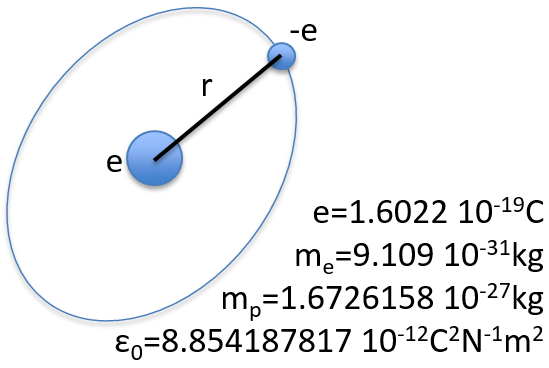In quantum mechanics, the system is described by the equation of Schrödinger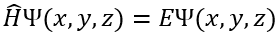Ψ is a wave function and Ĥ is the Hamiltonian, composed of one kinetic term and of one potential term.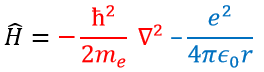The potential term is the potential of attraction between two opposite charges in the void (ϵ0 is the void permittivity).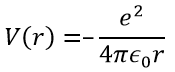The kinetic term describes the movement of the electron in orbit around the nucleus, where ћ=h/2π and the gradient ∇ is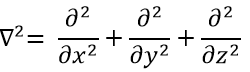in Cartesian coordinates. It is interesting to change for spherical coordinates. The expression looks more complex but allows to separate some expressions.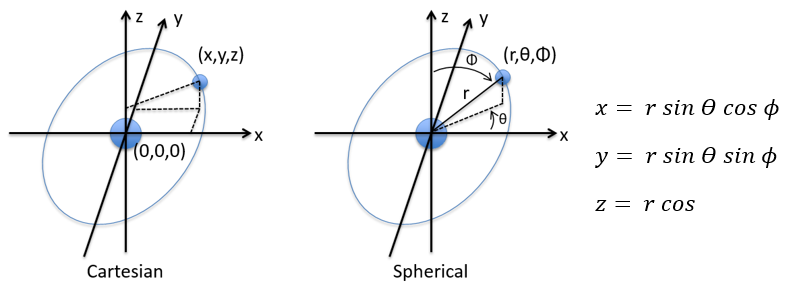Then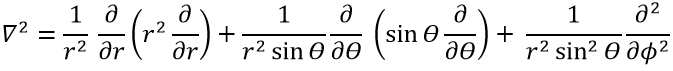This expression seems more complex than the previous one, but there will be one big advantage. The equation of Schrödinger becomes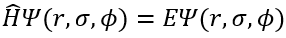This equation, developed, gives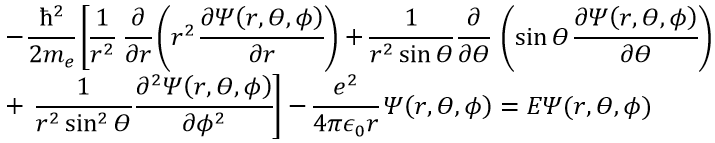As a result, the wave function can now be separated into one radial equation and one angular equation.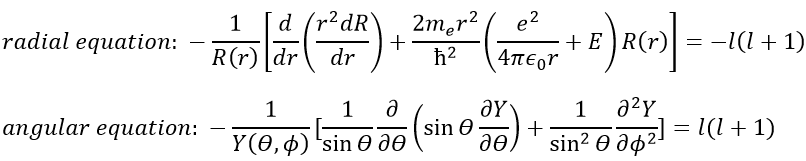The solution of the equations are directly related to the quantic number l. As a reminder, the quantic numbers n, l and m give the electronic configuration of the atoms with l between zero and n (O ≤ l ≤ n) and m between –l and l (–l ≤ m ≤ l). For instance if n=2, l can have a value of 1 or 0, giving 3 possible values for m: -1, 0 (twice), 1.  Fermi told us that we may put 2 electrons of opposite spin on each orbital. For the atoms, the orbitals are noted s, p, d, f, g,… for l=0, 1, 2, 3, 4,… preceded by the quantic number n.We will first try to solve the radial equation. To do that we multiply everything by ћ2/2mer2 and change the variable from R(r) to P(r)=rR(r).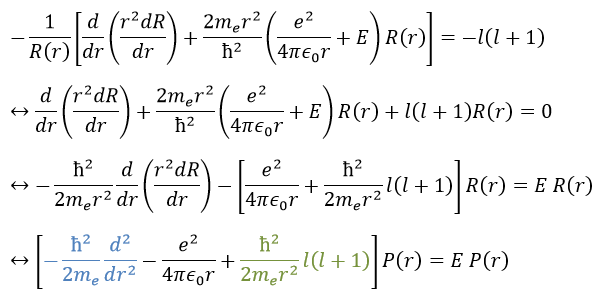There are three terms in the equation: the radial kinetic energy, the Coulomb potential and the centrifugal term. The equation is similar to the initial Hamiltonian but there is an additional centrifuge term. The potential term is now composed of a Coulomb term (potential of attraction) and of the centrifugal term.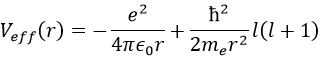If we consider the effective potential, we see that the centrifuge term repulses the electrons from the nucleus. The s orbitals (l=0) have no centrifugal term and their electrons are thus close to the nucleus. The other orbitals involve a positive centrifugal term moving the electrons away from the nucleus.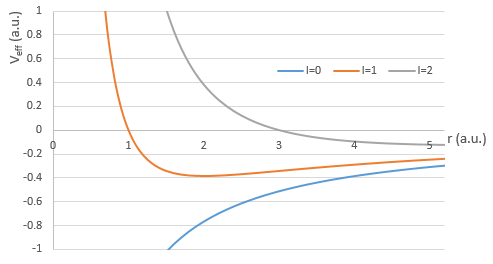The energy En of the orbitals depends on n and not on l or m.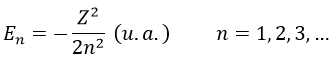It is proportional to 1/n2. The orbitals are thus closer and closer of each other when n increases. The energy is negative and tends to zero for n→∞, i.e. the ionisation. The energy is also proportional to the square of the atomic number. The energy is thus different for H or He+, or any atom with one single electron despite the similar structure.

The solution for the wave function is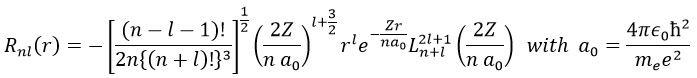The wave function Rnl(r) will thus depend on the quantic numbers n, l, the atomic number and the radius. In the case of s orbitals, the wave function is not equal to zero at r=0. Yet the density of probability is r2.Rnl(r)2 and there is thus no electron at the nucleus.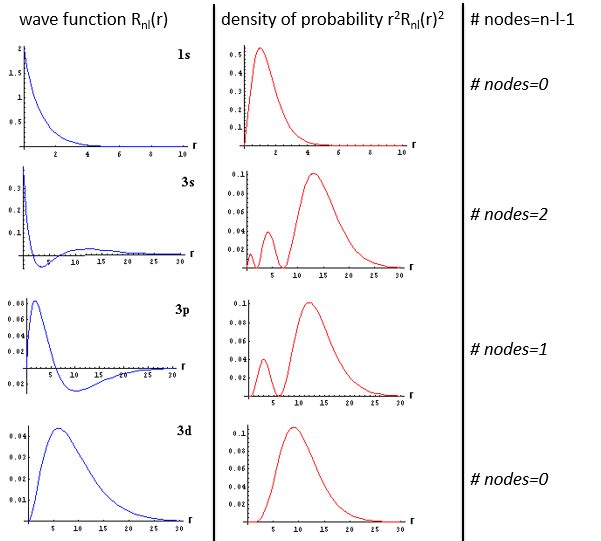As the density of probability is the square of something, the areas with a negative value of Rnl(r) can possess electrons. The points where the density of probability equals zero are called nodes and their number is equal to n-l-1. Note that there is thus no fixed radius for an electron but for its largest probability of presence and that even if the energy of the orbitals are equal, the electrons are not at the same distance from the nucleus. For monoelectronic atoms, the orbitals 3s and 3p are thus at the same energy. They are degenerated. It is the case for all the orbitals with a same value of n.

Now, let’s take a look at the angular wave function Y.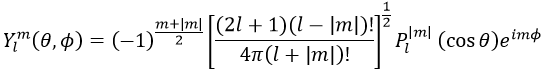Where Pl∣m∣(cos ) is the associated functions of Legendre. The solution here does not depend on n but on l and m. A few solutions are given below: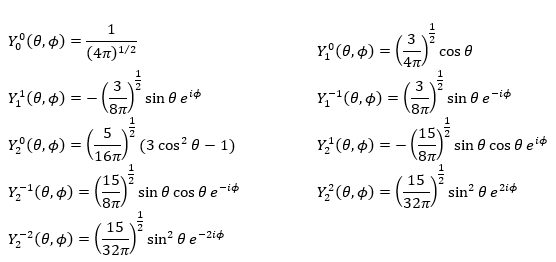The first angular wave function Y00 gives a sphere as it has no dependence on the angles and ϕ. The other functions have different shapes that depends on the angles.

To obtain atomic orbitals we just have to combine the angular and the radial wave functions. The orbitals s are spherical. There are 3 orbitals p because there are 3 possible values for m: -1, 0 or 1. The orbitals are identical but point in different directions. Actually it is a bit more complicated: the angular wave functions for m=-1 and 1 have an imaginary term that we get rid of by a change of coordinates, back to the Cartesian ones. We can do this change with a combination of the orbitals. We can draw the orbitals with the following technique: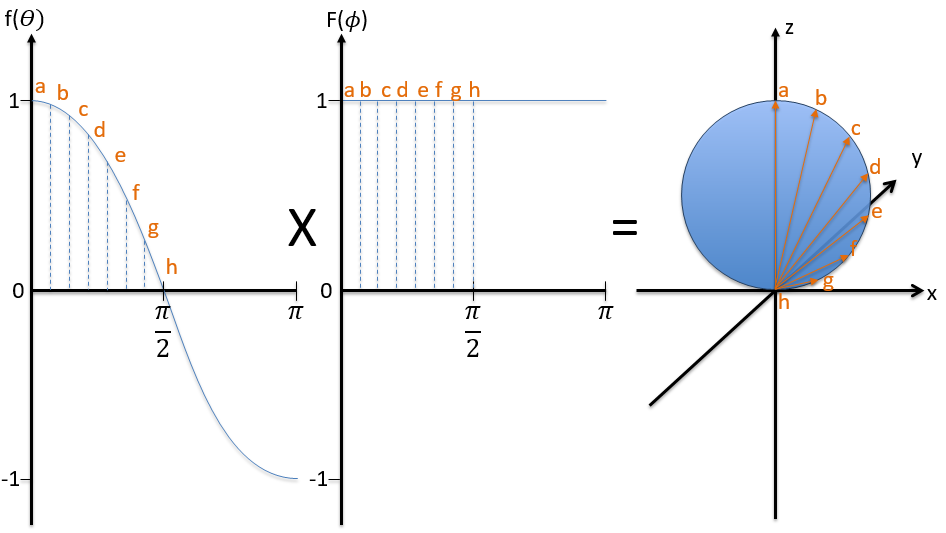First we take the functions that depend on one angle and draw their value as a function of the angle. For the orbital pz the functions are f()=cos and F(ϕ)=1. While F=1 for any angle, f goes from 1 to -1. The orbital is given by the multiplication of the two functions. F=constant means that the orbital is identical for any value of ϕ. The axis z is thus an axis of symmetry. is the angle in the z direction. When =0 (point a), we are on the axis z and the intensity is 1. When >0, we are not on the axis. The intensity is smaller than one so we draw a point at a smaller distance from the centre than the previous point. The intensity keeps decreasing to reach zero at =π/2 (point g), the intersection with the plane x=0, y=0. In fact, we just drew one sphere above the plane x=0, y=0. The same is done at the other side of the plane (π/2< <π). This part of the orbital has a negative sign because the function is negative (cos <0). We can repeat this method for the other directions.